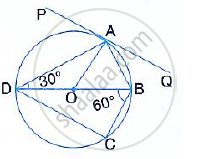Share

# In the Following Figure, Pq is the Tangent to the Circle at A, Db is the Diameter and O is the Centre of the Circle. If ∠Adb = 30° and ∠Cbd = 60°, Calculate: (I) ∠Qab, (Ii) ∠Pad, (Iii) ∠Cdb, - Mathematics

Course

#### Question

In the following figure, PQ is the tangent to the circle at A, DB is the diameter and O is the centre of the circle. If ∠ADB = 30° and ∠CBD = 60°, Calculate: ∠QAB#### Solution

PAQ is a tangent and AB is the chord.

∠ QAB = ∠ ADB = 30° (angles in the alternate segment)

Is there an error in this question or solution?